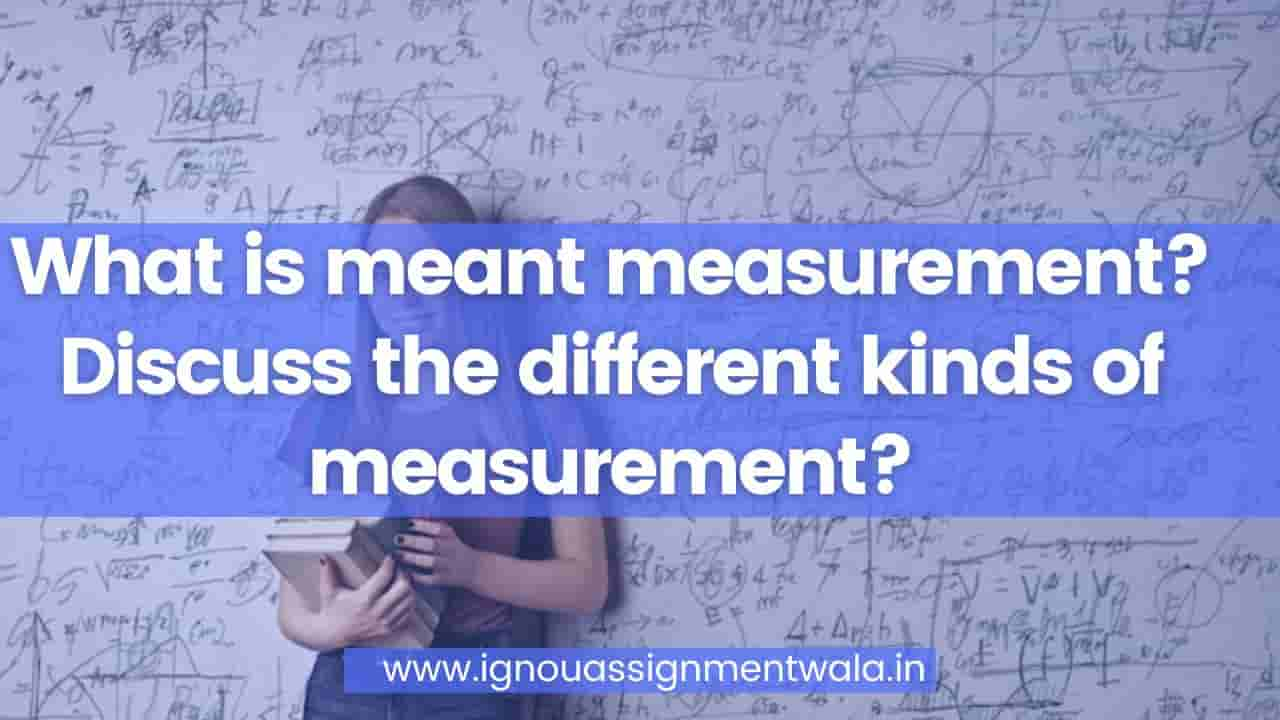## measurement

Measurement is the basic concept in the study of Mathematics and Science. Measurement quantifies the characteristics of an object or event, which we can compare with other things or events. Measurement is the most commonly used word, whenever we deal with the division of a quantity. Also, in taking a certain amount of things to accomplish a particular task. In our daily existence, we often come across different measurement types for length, weight, times, etc.

## Measurement Definition

Measurement is a technique in which the properties of an object are determined by comparing them to a standard quantity. Also, measurement is the essential metric to express any quantity of objects, things and events.

## Types of Measurement

There are some basic types of measurement variables that we deal with in several areas of mathematics. They are:

• Time
• Length
• Weight
• Volume
• Temperature

### Measurement Units

We have units of measurement for different types of measurement as mentioned above. Let’s have a look at the below units of measurement.

Time: Units for expressing time include seconds, minutes, hours, days, weeks, months, years, etc.

Length: Units for measuring length include millimetres, centimetres, meters, kilometres, etc.

Weight: Units for expressing the weight of certain objects include grams, kilograms, tons, etc.

Volume: Units for expressing volume include cm3, m3, litres, etc.

Temperature: The major units of temperature include centigrade and fahrenheit.